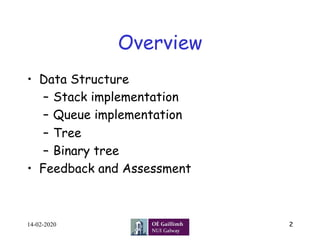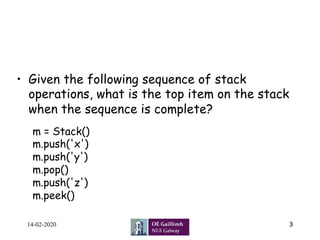Ce diaporama a bien été signalé.
Le téléchargement de votre SlideShare est en cours. ×
Publicité
Publicité
Publicité
Publicité
Publicité
Publicité
Publicité
Publicité
Publicité
Publicité
PublicitéChargement dans…3
×

## Consultez-les par la suite

1 sur 26 Publicité

# L 17 ct1120

Class lecture of Data Structure and Algorithms and Python.
Stack, Queue, Tree, Python, Python Code, Computer Science, Data, Data Analysis, Machine Learning, Artificial Intellegence, Deep Learning, Programming, Information Technology, Psuedocide, Tree, pseudocode, Binary Tree, Binary Search Tree, implementation, Binary search, linear search, Binary search operation, real-life example of binary search, linear search operation, real-life example of linear search, example bubble sort, sorting, insertion sort example, stack implementation, queue implementation, binary tree implementation, priority queue, binary heap, binary heap implementation

Class lecture of Data Structure and Algorithms and Python.
Stack, Queue, Tree, Python, Python Code, Computer Science, Data, Data Analysis, Machine Learning, Artificial Intellegence, Deep Learning, Programming, Information Technology, Psuedocide, Tree, pseudocode, Binary Tree, Binary Search Tree, implementation, Binary search, linear search, Binary search operation, real-life example of binary search, linear search operation, real-life example of linear search, example bubble sort, sorting, insertion sort example, stack implementation, queue implementation, binary tree implementation, priority queue, binary heap, binary heap implementation

Publicité
Publicité

### L 17 ct1120

1. 1. First Year BA (IT) CT1120 Algorithms Lecture 17 Dr. Zia Ush Shamszaman z.shamszaman1@nuigalway.ie 1 School of Computer Science, College of Science and Engineering 14-02-2020
2. 2. Overview •  Data Structure –  Stack implementation –  Queue implementation –  Tree –  Binary tree •  Feedback and Assessment 214-02-2020
3. 3. •  Given the following sequence of stack operations, what is the top item on the stack when the sequence is complete? 14-02-2020 3 m = Stack() m.push('x') m.push('y') m.pop() m.push('z') m.peek()
4. 4. •  Given the following sequence of stack operations, what is the top item on the stack when the sequence is complete? 14-02-2020 4 m = Stack() m.push('x') m.push('y') m.pop() m.push('z') m.peek()
5. 5. What this code is doing? 14-02-2020 5 stack = [] stack.append('a') stack.append('b') stack.append('c') print('Initial stack') print(stack) print('nElements poped from stack:') print(stack.pop()) print(stack.pop()) print(stack.pop()) print('nStack after elements are poped:') print(stack)
6. 6. Stack implementation code 14-02-2020 6 stack = [] stack.append('a') stack.append('b') stack.append('c') print('Initial stack') print(stack) print('nElements poped from stack:') print(stack.pop()) print(stack.pop()) print(stack.pop()) print('nStack after elements are poped:') print(stack) OUTPUT Initial stack ['a', 'b', 'c'] Elements poped from stack: c b a Stack after elements are poped: []
7. 7. What this code is doing? 14-02-2020 7 queue = [] queue.append('a') queue.append('b') queue.append('c') print("Initial queue") print(queue) print("nElements dequeued from queue") print(queue.pop(0)) print(queue.pop(0)) print(queue.pop(0)) print("nQueue after removing elements") print(queue)
8. 8. What this code is doing? 14-02-2020 8 queue = [] queue.append('a') queue.append('b') queue.append('c') print("Initial queue") print(queue) print("nElements dequeued from queue") print(queue.pop(0)) print(queue.pop(0)) print(queue.pop(0)) print("nQueue after removing elements") print(queue) Output Initial queue ['a', 'b', 'c'] Elements dequeued from queue a b c Queue after removing elements []
9. 9. Tree •  A Tree is a collection of elements called nodes. •  One of the node is distinguished as a root, along with a relation (“parenthood”) that places a hierarchical structure on the nodes. 14-02-2020 9
10. 10. Applications of Tree 14-02-2020 10 Main applications of trees include: 1. Manipulate hierarchical data. 2. Make information easy to search (see tree traversal). 3. Manipulate sorted lists of data. 4. As a workflow for compositing digital images for visual effects. 5. Router algorithms 6. Form of a multi-stage decision-making (see business chess).
11. 11. Binary Tree 14-02-2020 11 •  A tree whose elements have at most 2 children is called a binary tree. •  Since each element in a binary tree can have only 2 children, we typically name the left child and right child.
12. 12. Binary Tree 14-02-2020 12 •  A tree whose elements have at most 2 children is called a binary tree. •  Since each element in a binary tree can have only 2 children, we typically name the left child and right child. ((7+3)∗(5−2))
13. 13. Binary Tree 14-02-2020 13 •  A tree whose elements have at most 2 children is called a binary tree. •  Since each element in a binary tree can have only 2 children, we typically name the left child and right child. •  A Binary Tree node contains following parts. •  Data •  Pointer to left child •  Pointer to right child
14. 14. Python code of Binary Tree 14-02-2020 14 # A class that represents an individual node in a # Binary Tree class Node: def __init__(self,key): self.left = None self.right = None self.val = key # create root root = Node(1) ''' following is the tree after above statement 1 / None None''' root.left = Node(2); root.right = Node(3); ''' 2 and 3 become left and right children of 1 1 / 2 3 / / None None None None''’ root.left.left = Node(4); '''4 becomes left child of 2 1 / 2 3 / / 4 None None None / None None''' "__init__" is a reseved method in python classes. It is called as a constructor in object oriented terminology. This method is called when an object is created from a class and it allows the class to initialize the attributes of the class.
15. 15. Tree traversal 14-02-2020 15 Following are the generally used ways for traversing trees: •  Inorder: We recursively do an inorder traversal on the left subtree, visit the root node, and finally do a recursive inorder traversal of the right subtree. •  Preorder: We visit the root node first, then recursively do a preorder traversal of the left subtree, followed by a recursive preorder traversal of the right subtree. •  Postorder: We recursively do a postorder traversal of the left subtree and the right subtree followed by a visit to the root node.
16. 16. Tree traversal 14-02-2020 16 (a) Inorder (Left, Root, Right) : 4 2 5 1 3 (b) Preorder (Root, Left, Right) : 1 2 4 5 3 (c) Postorder (Left, Right, Root) : 4 5 2 3 1 Level Order Traversal : 1 2 3 4 5
17. 17. Tree traversal 14-02-2020 17 (a) Inorder (Left, Root, Right) : 4 2 5 1 3 (b) Preorder (Root, Left, Right) : 1 2 4 5 3 (c) Postorder (Left, Right, Root) : 4 5 2 3 1 Level Order Traversal : 1 2 3 4 5
18. 18. Tree traversal code 14-02-2020 18 # A class that represents an individual node in a Binary Tree class Node: def __init__(self,key): self.left = None self.right = None self.val = key # A function to do inorder tree traversal def printInorder(root): if root: # First recur on left child printInorder(root.left) # then print the data of node print(root.val), # now recur on right child printInorder(root.right) # A function to do postorder tree traversal def printPostorder(root): if root: # First recur on left child printPostorder(root.left) # the recur on right child printPostorder(root.right) # now print the data of node print(root.val), # A function to do preorder tree traversal def printPreorder(root): if root: # First print the data of node print(root.val), # Then recur on left child printPreorder(root.left) # Finally recur on right child printPreorder(root.right) # Driver code root = Node(1) root.left = Node(2) root.right = Node(3) root.left.left = Node(4) root.left.right = Node(5) print ("nPreorder traversal of binary tree is") printPreorder(root) print ("nInorder traversal of binary tree is") printInorder(root) print ("nPostorder traversal of binary tree is") printPostorder(root)
19. 19. Inorder traversal without recursion 14-02-2020 19 # A binary tree node class Node: # Constructor to create a new node def __init__(self, data): self.data = data self.left = None self.right = None # Iterative function for inorder tree traversal def inOrder(root): # Set current to root of binary tree current = root stack = [] # initialize stack done = 0 while True: # Reach the left most Node of the current Node if current is not None: # Place pointer to a tree node on the stack # before traversing the node's left subtree stack.append(current) current = current.left # BackTrack from the empty subtree and visit the Nod # at the top of the stack; however, if the stack is # empty you are done elif(stack): current = stack.pop() print(current.data, end=" ") # Python 3 printing # We have visited the node and its left # subtree. Now, it's right subtree's turn current = current.right else: break print() # Driver program to test above function """ Constructed binary tree is 1 / 2 3 / 4 5 """ root = Node(1) root.left = Node(2) root.right = Node(3) root.left.left = Node(4) root.left.right = Node(5) inOrder(root)
20. 20. Priority Queue 14-02-2020 20 •  A priority queue acts like a queue in that you dequeue an item by removing it from the front. •  In a priority queue the logical order of items inside a queue is determined by their priority. •  The highest priority items are at the front of the queue and the lowest priority items are at the back. •  When you enqueue an item on a priority queue, the new item may move all the way to the front.
21. 21. Binary Heaps 14-02-2020 21 •  A Heap is a special Tree-based data structure in which the tree is a complete binary tree. Generally, Heaps can be of two types: •  Max-Heap: The key present at the root node must be greatest among the keys present at all of it’s children. The same property must be recursively true for all sub-trees in that Binary Tree. •  Min-Heap: The key present at the root node must be minimum among the keys present at all of it’s children. The same property must be recursively true for all sub-trees in that Binary Tree.
22. 22. Binary Heap Operation 14-02-2020 22 The basic operations we will implement for our binary heap are as follows: •  BinaryHeap() creates a new, empty, binary heap. •  insert(k) adds a new item to the heap. •  findMin() returns the item with the minimum key value, leaving item in the heap. •  delMin() returns the item with the minimum key value, removing the item from the heap. •  isEmpty() returns true if the heap is empty, false otherwise. •  size() returns the number of items in the heap. •  buildHeap(list) builds a new heap from a list of keys.
23. 23. Next Lecture 14-02-2020 23
24. 24. •  Binary Heap Implementation •  Binary Search Tree 14-02-2020 24
25. 25. Useful Links •  http://www.csanimated.com/animation.php?t=Quicksort •  http://www.hakansozer.com/category/c/ •  http://www.nczonline.net/blog/2012/11/27/computer-science-in- javascript-quicksort/ •  http://www.sorting-algorithms.com/shell-sort •  https://www.tutorialspoint.com/ •  https://www.hackerearth.com/ •  www.khanacademy.org/computing/computer-science/algorithms 14-02-2020 25
26. 26. Feedback & Assessment 14-02-2020 26